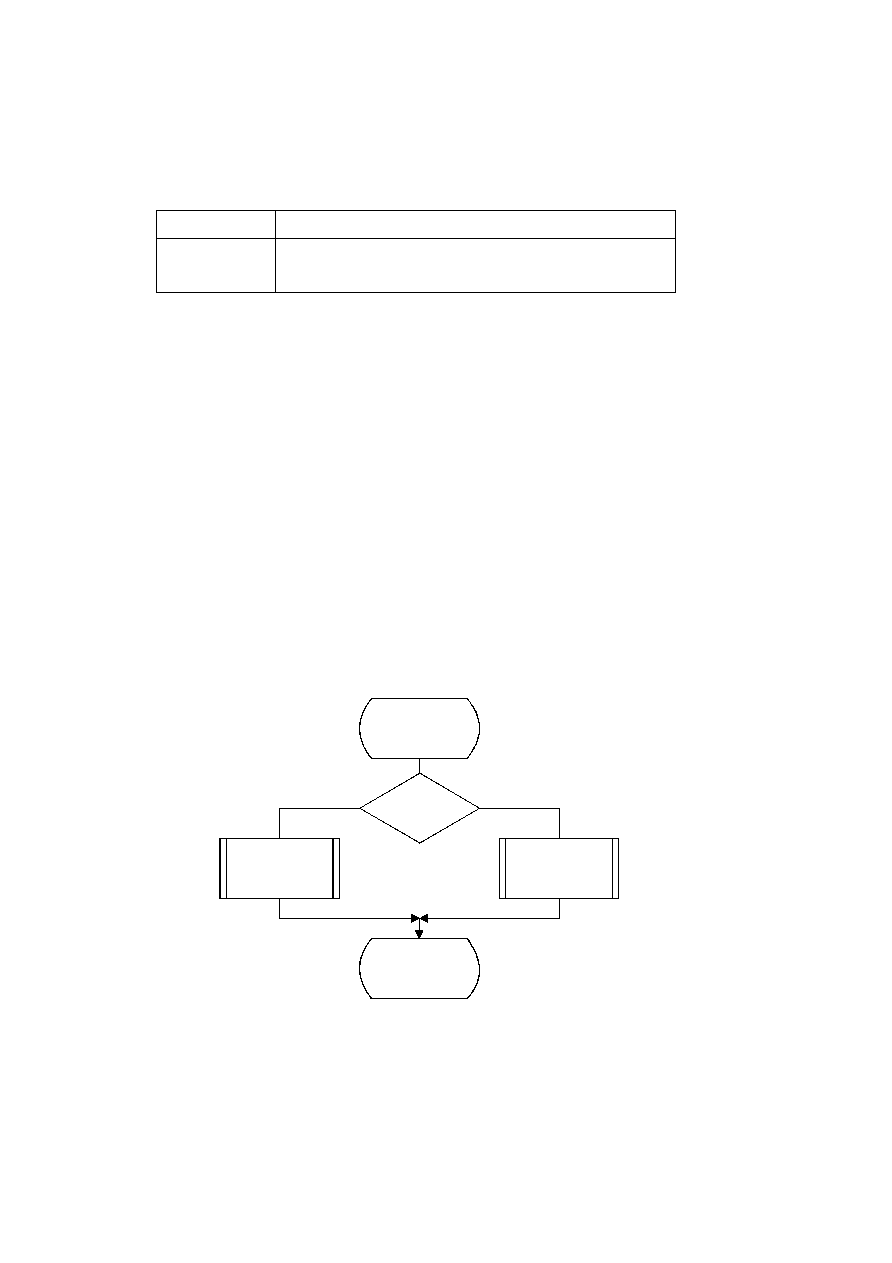ISO/IEC 10918-1 : 1993(E)
D.1.3
Encoder code register conventions
The flow charts in this annex assume the register structures for the encoder as shown in Table D.2.
Table D.2 ­ Encoder register connections
MSB
LSB
C-register
0000cbbb,
bbbbbsss,
xxxxxxxx,
xxxxxxxx
A-register
00000000,
00000000,
aaaaaaaa,
aaaaaaaa
The "a" bits are the fractional bits in the A-register (the current probability interval value) and the "x" bits are the
fractional bits in the code register. The "s" bits are optional spacer bits which provide useful constraints on carry-over, and
the "b" bits indicate the bit positions from which the completed bytes of data are removed from the C-register. The "c" bit
is a carry bit. Except at the time of initialization, bit 15 of the A-register is always set and bit 16 is always clear (the LSB
is bit 0).
These register conventions illustrate one possible implementation. However, any register conventions which allow
resolution of carry-over in the encoder and which produce the same entropy-coded segment may be used. The handling of
carry-over and the byte stuffing following X'FF' will be described in a later part of this annex.
D.1.4
Code_1(S) and Code_0(S) procedures
When a given binary decision is coded, one of two possibilities occurs ­ either a 1-decision or a 0-decision is coded.
Code_1(S) and Code_0(S) are shown in Figures D.1 and D.2. The Code_1(S) and Code_0(S) procedures use probability
estimates with a context-index S. The context-index S is determined by the statistical model and is, in general, a function
of the previous coding decisions; each value of S identifies a particular conditional probability estimate which is used in
encoding the binary decision.
TISO1800-93/d039
Code_1(S)
No
Yes
MPS(S) = 1
?
Code_LPS(S)
Code_MPS(S)
Done
Figure D.1 ­ Code_1(S) procedure
Figure D.1 [D39], = 9 cm = 352.%
56
CCITT Rec. T.81 (1992 E)